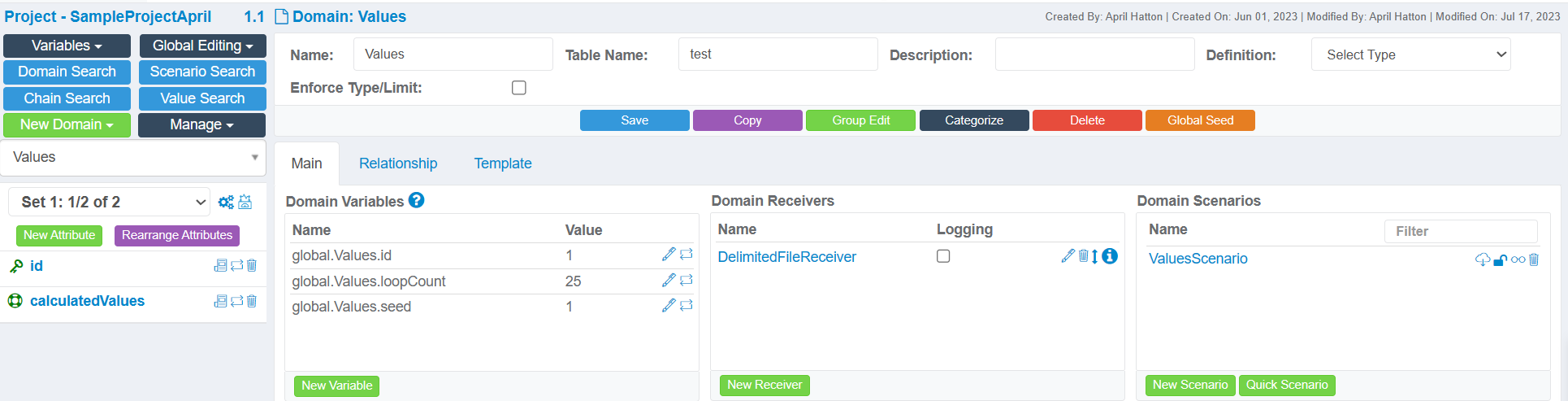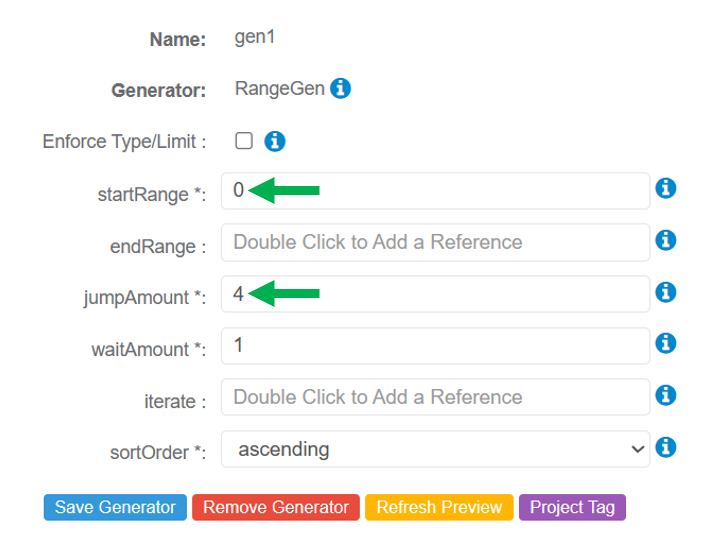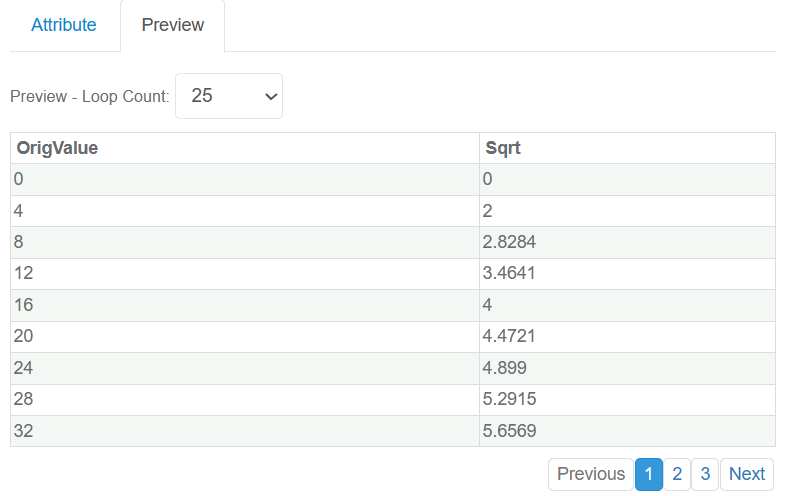# Description

The MathGen Generator performs 17 common math functions on a constant or referenced value (linked Generator or Attribute).

Note: Please note that these are Java Math Methods.

## Generator Parameters

The following parameters may be configured for the MathGen Generator. Items with an asterisk* are required.

• reference* - Defines a constant value or referenced value (linked Generator or Attribute) containing the value to perform the calculation.

• function* - Defines the function to use on the constant or referenced value. More information about each function is provided in Math Functions Reference Table.

• decimalFormat*- Defines the decimal number format in the generated result.## Use Case Example - Generate Square Root of Patterned Numbers

This example will show how the MathGen Generator can be used to generate the square root of numbers generated by a linked Generator within the same Attribute.

### Project Setup

A Project has been created with the default Project Version and a Values Domain. The Domain contains two Attributes: id and calculatedValues.The calculatedValues Attribute contains two linked Generators:

• RangeGen - Generates the patterned numbers that will be used to calculate the square root for each record.
• MathGen - Generates the square root of the referenced value for each record.### RangeGen Generator

The following parameters have been configured:

• StartRange = 0
• JumpAmount = 4The first generated value will be 0 and then increase by 4 for each subsequent record. An example is shown below:### MathGen Generator

The MathGen Generator will reference the values generated by the RangeGen Generator and use them to calculate the square root for each record.

The configuration will appear as shown below, and the following parameter values have been changed:

• reference = references Gen 1 (RangeGen)
• function = sqrt### Sample Output

The sample set of data below shows the original referenced value and the calculated square root of that value:## Math Functions Reference Table

The following math functions are available with the MathGen Generator.

### Example

Absolute ValueabsGenerates the positive value of the reference value. Referenced Value = -6
Generated Value = 6
Arc Cosine
acos
The inverse of the Cosine function. Generates the arc cosine of an angle between 0.0 and pi (3.14159...).

Note: If the absolute value is greater than 1, then the result is NaN.
Referenced Value = 0.0
Generated Value = 1.5707963
Arc SineasinThe inverse of the Sine function. Generates the arc sine of an angle between -pi/2 and pi/2.

Note: If the absolute value is greater than 1, then the result is NaN.

Note: If the referenced value is 0, the generated value is zero with the same sign as the referenced value.
Referenced Value = 0.5
Generated Value = 0.523599
Arc TangentatanThe inverse of the Tangent function. Generates the arc tangent of an angle between -pi/2 through pi/2.

Note: If the referenced value is 0, the generated value is zero with the same sign as the referenced value.
Referenced Value = 1.5
Generated Value = 0.98279372
CeilingceilGenerates a value that is the next highest integer of the given number.
Referenced Value = 11.5
Generated Vaue = 12
Cube RootcbrtGenerates the cube root of a double value. The value is cubed or multiplied by itself three times.

Note: If the referenced value is 0, the generated value is zero with the same sign as the referenced value.
Referenced Value = 1000
Generated Value = 10
CosinecosGenerates the trigonometric cosine of an angle.
Referenced Value (in radians) = 1.04719755
Generated Value = 0.5000000
DegreesdegreesThe referenced value is interpreted as radians. Generates approximately equivalent value in degrees. Referenced Value = 0.78539816
Generated Value = 45
ExponentialexpGenerates the number e raised to the power of a double value.
Referenced Value = 1
Generated Value =  2.718281828459045
FloorfloorGenerates the largest mathematical integer that is less than or equal to the referenced value.

Note: If the referenced value is a positive or negative 0, the generated value will be the same.
Referenced Value = 11.5
Generated Value = 11
LogarithmlogGenerates the natural logarithm (base e) of a double value as a parameter.

Note: If the referenced value is a positive or negative zero, the generated value will be negative infinity.
Referenced Value = 10
Generated Value = 2.30258509
Logarithm Base 10log10Generates the base 10 logarithmic value of a given double value as a parameter.

Note: If the referenced value is less than 0, the generated value will be NaN.

Note: If the referenced value is a positive or negative zero, the generated value will be negative infinity.
Referenced Value = 100
Generated Value = 2
Referenced Value = 45
Generated Value = 0.78539816
RoundroundThe number will be rounded to the nearest whole number based on the first decimal place value (tenths).
Referenced Value = 12.784537
Generated Value = 13
Square RootsqrtGenerates the square root of the entered or referenced value.

Note: If the referenced value is negative, the generated value will be NaN.
Referenced Value = 9
Generated Value = 3
SinesinGenerates the trigonometry sine of an angle between 0.0 and pi 3.14159...). The referenced value should be radians.

The generated value will be between -1 and 1.

Note: If the referenced value is a positive or negative 0, the generated value will be zero with the same sign as the referenced value.
Referenced Value =  0.523599
Generated Value = 0.5
TangenttanCalculates the tangent based on the given value(s). The reference value should be radians.

Note: If the referenced value is a positive or negative 0, the generated value will be zero with the same sign as the referenced value.
Referenced Value = 0.98279372
Generated Value = 1.5